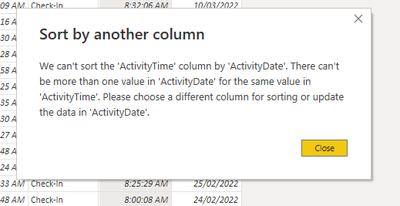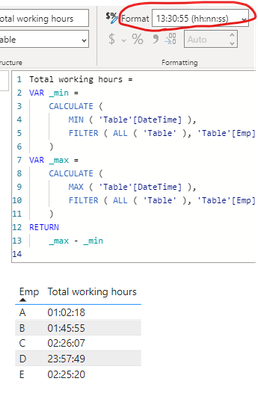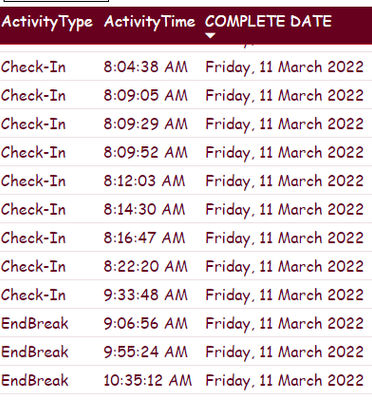cancel
Showing results for
Did you mean:Helper I

## SORTING AS PER SPECIFIC COLUMN3 REPLIES 3Microsoft

Hi @Neha_Shukla ,

I added a few rows of date and Emp columns to your original table.

1.Merge Complete Date and ActivityTime columns, Modify the date type to Datetime. Then sort directly.

``````DateTime =
CONVERT ( 'Table'[Complete Date] & " " & 'Table'[ActivityTime], DATETIME )``````2.Create a measure and group by max and min. Change the format.

``````Total working hours =
VAR _min =
CALCULATE (
MIN ( 'Table'[DateTime] ),
FILTER ( ALL ( 'Table' ), 'Table'[Emp] = MAX ( 'Table'[Emp] ) )
)
VAR _max =
CALCULATE (
MAX ( 'Table'[DateTime] ),
FILTER ( ALL ( 'Table' ), 'Table'[Emp] = MAX ( 'Table'[Emp] ) )
)
RETURN
_max - _min``````Best Regards,
Jinwei Li
If this post helps, then please consider Accept it as the solution to help the other members find it more quickly.Super User

@Neha_Shukla , You have two value which is getting the same sort of order that is not allowed

Something like this

 Column Sort A 1 B 1 C 2

That is not allowed

How to Create Sort Column and Solve Related Errors:Helper I1. I want to sort out activity time column as per latest time entry.

On activity type column there are values containing ( check - in , lunch - time, lunch- over, break- time, end- break , check-out)

2. AS per this activity which are done by employee I want to find out total working hours.